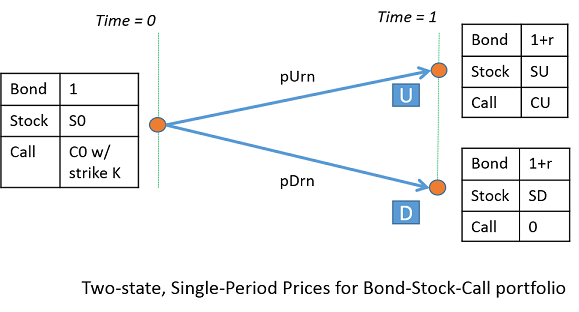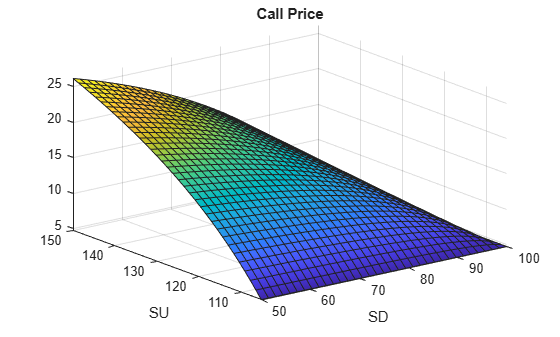# Explore Single-Period Asset Arbitrage

This example explores basic arbitrage concepts in a single-period, two-state asset portfolio. The portfolio consists of a bond, a long stock, and a long call option on the stock.

It uses these Symbolic Math Toolbox™ functions:

This example symbolically derives the risk-neutral probabilities and call price for a single-period, two-state scenario.### Define Parameters of the Portfolio

Create the symbolic variable `r` representing the risk-free rate over the period. Set the assumption that `r` is a positive value.

`syms r positive`

Define the parameters for the beginning of a single period, `time = 0`. Here `S0` is the stock price, and `C0` is the call option price with strike, `K`.

`syms S0 C0 K positive`

Now, define the parameters for the end of a period, `time = 1`. Label the two possible states at the end of the period as U (the stock price over this period goes up) and D (the stock price over this period goes down). Thus, `SU` and `SD` are the stock prices at states U and D, and `CU` is the value of the call at state U. Note that $SD<=K<=SU$.

`syms SU SD CU positive`

The bond price at `time = 0` is 1. Note that this example ignores friction costs.

Collect the prices at `time = 0` into a column vector.

`prices = [1 S0 C0]'`
```prices =  $\left(\begin{array}{c}1\\ {S}_{0}\\ {C}_{0}\end{array}\right)$```

Collect the payoffs of the portfolio at `time = 1` into the `payoff` matrix. The columns of `payoff` correspond to payoffs for states D and U. The rows correspond to payoffs for bond, stock, and call. The payoff for the bond is `1 + r`. The payoff for the call in state D is zero since it is not exercised (because $SD<=K$).

`payoff = [(1 + r), (1 + r); SD, SU; 0, CU]`
```payoff =  $\left(\begin{array}{cc}r+1& r+1\\ \mathrm{SD}& \mathrm{SU}\\ 0& \mathrm{CU}\end{array}\right)$```

`CU` is worth `SU - K` in state U. Substitute this value in `payoff`.

`payoff = subs(payoff, CU, SU - K)`
```payoff =  $\left(\begin{array}{cc}r+1& r+1\\ \mathrm{SD}& \mathrm{SU}\\ 0& \mathrm{SU}-K\end{array}\right)$```

### Solve for Risk-Neutral Probabilities

Define the probabilities of reaching states U and D.

`syms pU pD real`

Under no-arbitrage, `eqns == 0` must always hold true with positive `pU` and `pD`.

`eqns = payoff*[pD; pU] - prices`
```eqns =  $\left(\begin{array}{c}\mathrm{pD} \left(r+1\right)+\mathrm{pU} \left(r+1\right)-1\\ \mathrm{SD} \mathrm{pD}-{S}_{0}+\mathrm{SU} \mathrm{pU}\\ -{C}_{0}-\mathrm{pU} \left(K-\mathrm{SU}\right)\end{array}\right)$```

Transform equations to use risk-neutral probabilities.

```syms pDrn pUrn real; eqns = subs(eqns, [pD; pU], [pDrn; pUrn]/(1 + r))```
```eqns =  $\left(\begin{array}{c}\mathrm{pDrn}+\mathrm{pUrn}-1\\ \frac{\mathrm{SD} \mathrm{pDrn}}{r+1}-{S}_{0}+\frac{\mathrm{SU} \mathrm{pUrn}}{r+1}\\ -{C}_{0}-\frac{\mathrm{pUrn} \left(K-\mathrm{SU}\right)}{r+1}\end{array}\right)$```

The unknown variables are `pDrn`, `pUrn`, and `C0`. Transform the linear system to a matrix form using these unknown variables.

`[A, b] = equationsToMatrix(eqns, [pDrn, pUrn, C0]')`
```A =  $\left(\begin{array}{ccc}1& 1& 0\\ \frac{\mathrm{SD}}{r+1}& \frac{\mathrm{SU}}{r+1}& 0\\ 0& -\frac{K-\mathrm{SU}}{r+1}& -1\end{array}\right)$```
```b =  $\left(\begin{array}{c}1\\ {S}_{0}\\ 0\end{array}\right)$```

Using `linsolve`, find the solution for the risk-neutral probabilities and call price.

`x = linsolve(A, b)`
```x =  $\left(\begin{array}{c}\frac{{S}_{0}-\mathrm{SU}+{S}_{0} r}{\mathrm{SD}-\mathrm{SU}}\\ -\frac{{S}_{0}-\mathrm{SD}+{S}_{0} r}{\mathrm{SD}-\mathrm{SU}}\\ \frac{\left(K-\mathrm{SU}\right) \left({S}_{0}-\mathrm{SD}+{S}_{0} r\right)}{\left(\mathrm{SD}-\mathrm{SU}\right) \left(r+1\right)}\end{array}\right)$```

### Verify the Solution

Verify that under risk-neutral probabilities, `x(1:2)`, the expected rate of return for the portfolio, `E_return` equals the risk-free rate, `r`.

```E_return = diag(prices)\(payoff - [prices,prices])*x(1:2); E_return = simplify(subs(E_return, C0, x(3)))```
```E_return =  $\left(\begin{array}{c}r\\ r\\ r\end{array}\right)$```

### Test for No-Arbitrage Violations

As an example of testing no-arbitrage violations, use the following values: `r = 5%`, `S0 = 100`, and `K = 100`. For `SU < 105`, the no-arbitrage condition is violated because `pDrn = xSol(1)` is negative (`SU >= SD`). Further, for any call price other than `xSol(3)`, there is arbitrage.

`xSol = simplify(subs(x, [r,S0,K], [0.05,100,100]))`
```xSol =  $\left(\begin{array}{c}-\frac{\mathrm{SU}-105}{\mathrm{SD}-\mathrm{SU}}\\ \frac{\mathrm{SD}-105}{\mathrm{SD}-\mathrm{SU}}\\ \frac{20 \left(\mathrm{SD}-105\right) \left(\mathrm{SU}-100\right)}{21 \left(\mathrm{SD}-\mathrm{SU}\right)}\end{array}\right)$```

### Plot Call Price as a Surface

Plot the call price, `C0 = xSol(3)`, for `50 <= SD <= 100` and `105 <= SU <= 150`. Note that the call is worth more when the "variance" of the underlying stock price is higher for example, `SD = 50, SU = 150`.

```fsurf(xSol(3), [50,100,105,150]) xlabel SD ylabel SU title 'Call Price'```### Reference

`Advanced Derivatives, Pricing and Risk Management: Theory, Tools and Programming Applications` by Albanese, C., Campolieti, G.

## Support

#### Mathematical Modeling with Symbolic Math Toolbox

Get examples and videos